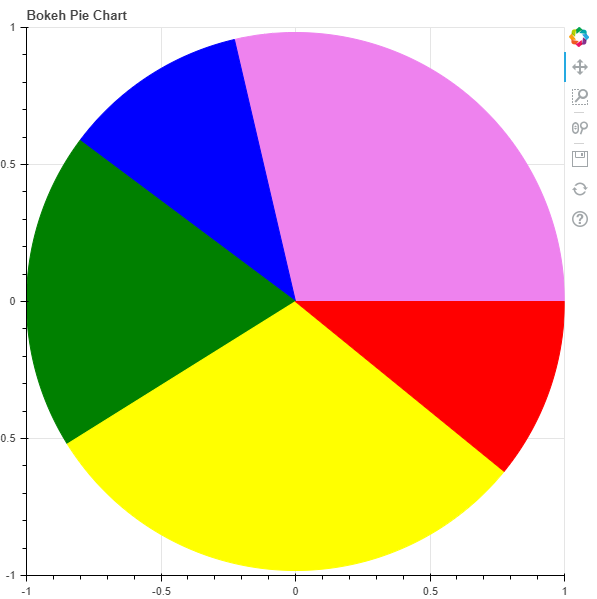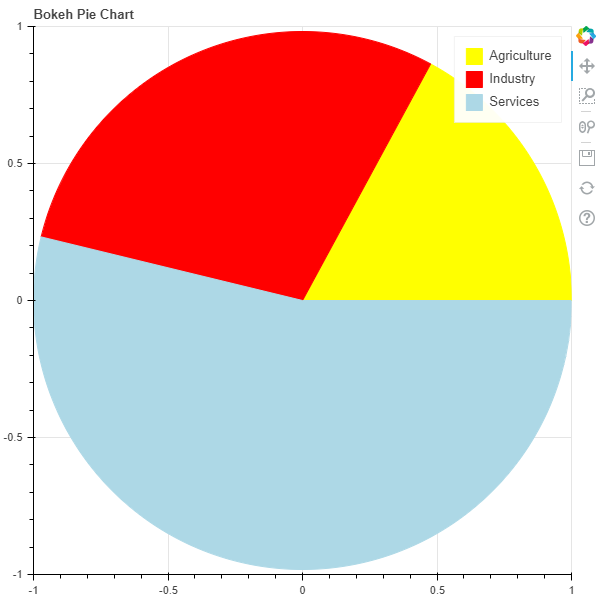GeeksforGeeks App
Open AppBrowser
Continue

# Python Bokeh – Making a Pie Chart

Bokeh is a Python interactive data visualization. It renders its plots using HTML and JavaScript. It targets modern web browsers for presentation providing elegant, concise construction of novel graphics with high-performance interactivity.

Let us see how to plot a pie chart in Bokeh. Does not provide us a module to directly plot a pie chart but we can use the wedge glyphs to make the pie chart. Wedges can be plotted using the `bokeh.plotting.figure.wedge()` function.

In the `wedge()` function, the primary parameters are the `x` and `y `coordinates of the wedge, the `radius`, the `start_angle `and the `end_angle` of the wedge. In order to plot the wedges in such a way that they look like a pie chart, the `x`, `y`, and `radius `parameters of all the wedges will be the same. We will only adjust the `start_angle` and the `end_angle`.

Example 1 :

 `# importing the modules``from` `bokeh.plotting ``import` `figure, output_file, show`` ` `# file to save the model ``output_file(``"gfg.html"``) ``          ` `# instantiating the figure object ``graph ``=` `figure(title ``=` `"Bokeh Pie Chart"``) `` ` `# center of the pie chart``x ``=` `0``y ``=` `0`` ` `# radius of the glyphs``radius ``=` `1`` ` `# starting angle values``start_angle ``=` `[``0``, ``1.8``, ``2.5``,``               ``3.7``, ``5.6``]`` ` `# ending angle values``end_angle ``=` `[``1.8``, ``2.5``, ``3.7``,``             ``5.6``, ``0``]`` ` `# color of the wedges``color ``=` `[``"violet"``, ``"blue"``, ``"green"``,``          ``"yellow"``, ``"red"``]`` ` `# plotting the graph``graph.wedge(x, y, radius,``            ``start_angle,``            ``end_angle,``            ``color ``=` `color)`` ` `# displaying the graph``show(graph)`

Output :Example 2 : In his example we will be visualizing some data. In the data we are provided with the Share of sectors in GVA during 2017-18 in India. The shares are :

```Agriculture - 17.1%
Industry - 29.1%
Services - 53.8%
```

To find the `start_angle `and the `end_angle `we will need to convert the percentages into radians using the following formula :

`math.radians((percent / 100) * 360)`

 `# importing the modules``from` `bokeh.plotting ``import` `figure, output_file, show``import` `math`` ` `# file to save the model ``output_file(``"gfg.html"``) ``          ` `# instantiating the figure object ``graph ``=` `figure(title ``=` `"Bokeh Pie Chart"``) `` ` `# name of the sectors``sectors ``=` `[``"Agriculture"``, ``"Industry"``, ``"Services"``]`` ` `# % tage weightage of the sectors``percentages ``=` `[``17.1``, ``29.1``, ``53.8``]`` ` `# converting into radians``radians ``=` `[math.radians((percent ``/` `100``) ``*` `360``) ``for` `percent ``in` `percentages]`` ` `# starting angle values``start_angle ``=` `[math.radians(``0``)]``prev ``=` `start_angle[``0``]``for` `i ``in` `radians[:``-``1``]:``    ``start_angle.append(i ``+` `prev)``    ``prev ``=` `i ``+` `prev`` ` `# ending angle values``end_angle ``=` `start_angle[``1``:] ``+` `[math.radians(``0``)]`` ` `# center of the pie chart``x ``=` `0``y ``=` `0`` ` `# radius of the glyphs``radius ``=` `1`` ` `# color of the wedges``color ``=` `[``"yellow"``, ``"red"``, ``"lightblue"``]`` ` `# plotting the graph``for` `i ``in` `range``(``len``(sectors)):``    ``graph.wedge(x, y, radius,``                ``start_angle ``=` `start_angle[i],``                ``end_angle ``=` `end_angle[i],``                ``color ``=` `color[i],``                ``legend_label ``=` `sectors[i])`` ` `# displaying the graph``show(graph)`

Output :My Personal Notes arrow_drop_up